Courses

# UPSC CSE Prelims Paper 2 (CSAT) Mock Test - 10 (Oct 4, 2021)

## 80 Questions MCQ Test UPSC CSE Prelims 2021 Mock Test Series | UPSC CSE Prelims Paper 2 (CSAT) Mock Test - 10 (Oct 4, 2021)

Description
This mock test of UPSC CSE Prelims Paper 2 (CSAT) Mock Test - 10 (Oct 4, 2021) for UPSC helps you for every UPSC entrance exam. This contains 80 Multiple Choice Questions for UPSC UPSC CSE Prelims Paper 2 (CSAT) Mock Test - 10 (Oct 4, 2021) (mcq) to study with solutions a complete question bank. The solved questions answers in this UPSC CSE Prelims Paper 2 (CSAT) Mock Test - 10 (Oct 4, 2021) quiz give you a good mix of easy questions and tough questions. UPSC students definitely take this UPSC CSE Prelims Paper 2 (CSAT) Mock Test - 10 (Oct 4, 2021) exercise for a better result in the exam. You can find other UPSC CSE Prelims Paper 2 (CSAT) Mock Test - 10 (Oct 4, 2021) extra questions, long questions & short questions for UPSC on EduRev as well by searching above.
QUESTION: 1

### Two boys, who study in a school, start walking to their homes from their school at 1 pm. The first boy, whose home is 5 km away from the school walks at a speed of 5 km/hr and the second boy whose home is 8 km away from the school walks at a speed of 6 km/hr. If their homes are in directly opposite directions, what will be the distance between the two boys at 2:10 pm?

Solution:

At the beginning, both the boys walk away from each other. After one hour, first boy reaches home and his distance from school is 5 km. This distance does not change after 2 pm.
The second boy walks at the speed of 6 km per hour for the entire 1 hour 10 min.
Distance covered by second boy in 1 hour 10 min = 7 km.
So, Total distance between the boys at 2:10 pm = 7 + 5 = 12 km.

QUESTION: 2

### Number of mobiles produced by Xiaomi Market share of bikes for year 2018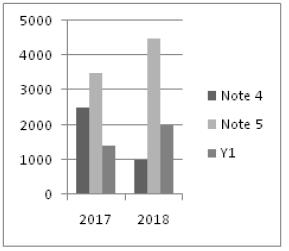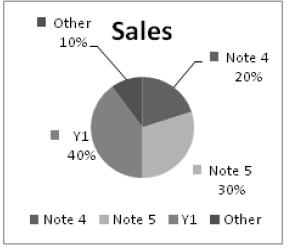If Xiaomi Company wants all its Y1 which are produced in 2017 and 2018 to be sold then what should have been the number of Y1 produced in 2018?

Solution:

We don’t know the market share of 2017. We can’t find the number of Y1 sold in year
2017.

QUESTION: 3

### Number of mobiles produced by Xiaomi Market share of bikes for year 2018If in 2018 Note 3 accounts for 8% of market share, what percent of others category does it account for?

Solution:

x% of 10% of total = 8% of total
x= (8×100)/10=80%

QUESTION: 4

Facing towards the South, who is just left to Ramakant in a row of the boys?
Statement-1. Suresh is just right of Chandrakant and he is fourth to the left of Ramakant.
Statement-2. Suresh is third to the left of Ramakant and Naresh is second to the left of Suresh.

Solution:

From Statement I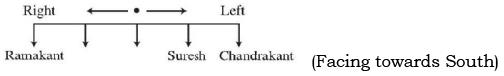Hence, we cannot determine who is right of Ramakant.
From Statement (2),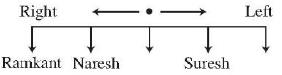Hence, Naresh is sitting left of Ramakant.

QUESTION: 5

A car is stolen at 2:30 pm and the thief runs away with the car driving at a speed of 60 km/hr. The owner discovers about the theft at 3 pm. He sets off in another car at a speed of 75 km/hr. If the owner drives on the correct path directly behind the thief, he would be able to catch the thief at:

Solution:

Assume that the thief is overtaken x hrs after 2.30 pm
When the car is overtaken, the distance covered by the owner and the thief is the same.
Distance covered by the thief in x hrs = distance covered by the owner in (x-0.5)hr
So, 60x = 75 (x- 0.5)
Or x = 2.5 hr
Thief is caught at 2.30 pm + 2.5 hr = 5 pm

QUESTION: 6

Number of mobiles produced by Xiaomi Market share of bikes for year 2018If in 2019 Galaxy 9 enters the market and is able to capture 5% of the 50,000 market then what is the percentage difference between market share of Galaxy 9 and Note 4? (Assuming percentage market share of Xiaomi mobiles and others is the same as in the previous year)

Solution:

% market share of Galaxy 9 = 5% and percentage share of Note 4 = (20/100) × 95 = 19%
Required percentage difference = (19 – 5) % = 14%

QUESTION: 7

Number of mobiles produced by Xiaomi Market share of bikes for year 2018If the market share of others remains the same, what is the total number of One plus mobiles sold in 2018, if One plus enters as a new player in market in 2018 and has the same market share as Xiaomi?

Solution:

Others have 10% share.
One plus has 45% share.
Xiaomi has 45% share (as percentage shares of Xiaomi and One plus are the same). and 45% of 150000 = 67500.

QUESTION: 8

Directions: Symbols @, %, #, \$, © are used with different meanings as explained below: ‘P @ Q’ means ‘P is not greater than Q’. ‘P % Q’ means ‘P is neither greater than nor equal to Q’. ‘P # Q’ means ‘P is neither smaller than nor equal to Q’. ‘P \$ Q’ means ‘P is neither smaller than nor greater than Q’. ‘P © Q’ means ‘P is not smaller than Q’.
Three statements showing relationships have been given, which are followed by two conclusions (1) and (2). Assuming that the given statements are true, find out which conclusions(s) is/are definitely true.

Q. Statements: J # P, P @ B, B % H.
Conclusions:
1. J @ B
2. J % H

Solution:

J # P = J > P,
P @ B = P ≤ B,
B % H =B < H
∴ J > P ≤ B < H
1. J @ B _ J < B (False)
2. J % H _ J < H (False)

QUESTION: 9

A class contains N students, having roll numbers 1,2,3....N. On a particular day only 50 students turn up for the class. The average of the roll numbers of the students present on that day is 38. A teacher punishes two students and sends them out of the class. The average of the roll numbers of the students who are left in the class drops to 37.5. Choose the correct statement from the options given below.

Solution:

Total of 50 roll numbers= 50 × 38 = 1900
Total of 48 roll numbers = 37.5 × 48 = 1800
Sum of the roll numbers of the students who have been sent out = 1900 – 1800 = 100.

QUESTION: 10

Expenditures of a Company (in Lakh Rupees) per Annum Over the given Years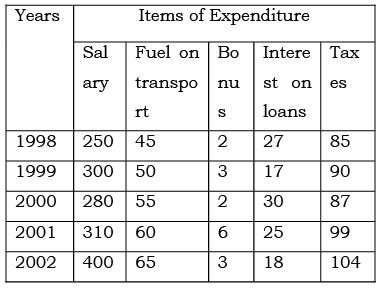The total amount of bonus paid by the company during the given period is approximately what percent of the total amount of salary paid during this period?

Solution:

Total bonus paid = 16 Total salary = 1540 Percentage = (16/1540) X 100 = 1.03%

QUESTION: 11

Expenditures of a Company (in Lakh Rupees) per Annum Over the given YearsThe ratio between the total expenditure on Taxes for all the years and the total expenditure on Fuel and Transport for all the years respectively is approximate?

Solution:

Total spent on taxes = 465
Total spent on fuel & transport = 275
Ratio = 465/275 = 93/55 = 93 : 55

QUESTION: 12

Directions for: Examine the information given in the following paragraph and answer the items there follow:

A school is celebrating Sport week in which five games viz. Cricket, Badminton, Tennis, Football and Volleyball are to be held in five different days in a week from Monday to Friday. Only one sport can be played on each day. Cricket cannot be played on Tuesday. Players for Badminton is available only on Tuesday. Volleyball is played immediately after the day of Cricket. Football has to played immediately before the day of Cricket.

Q. Which sport is to be played on Monday?

Solution:

Badminton is on Tuesday. Cricket is on Thursday because Volleyball has to be immediately after that (Friday) and Football immediately before Cricket (Wednesday). Remaining, Tennis subject is on Monday.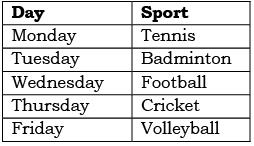QUESTION: 13

Directions for: Examine the information given in the following paragraph and answer the items there follow:

A school is celebrating Sport week in which five games viz. Cricket, Badminton, Tennis, Football and Volleyball are to be held in five different days in a week from Monday to Friday. Only one sport can be played on each day. Cricket cannot be played on Tuesday. Players for Badminton is available only on Tuesday. Volleyball is played immediately after the day of Cricket. Football has to played immediately before the day of Cricket.

Q. Which sport is scheduled between Tennis and Football?

Solution:

Badminton is on Tuesday. Cricket is on Thursday because Volleyball has to be immediately after that (Friday) and Football immediately before Cricket (Wednesday). Remaining, Tennis subject is on Monday.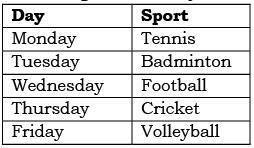QUESTION: 14

Five years ago, the average age of a family, which consisted of three members, was 27 years. The family now consists of four members as a baby was born recently. The current average age of the family is 25 years. How many years ago was the baby born?

Solution:

Average age of the family 5 years ago (3 members) = 27 years
Sum of the ages of the 3 older members now= (27 + 5) × 3 = 96 years
Average age of the family now (4 members) = 25 years
Sum of the ages of the 4 numbers of the family now = 25 ×4 = 100 years
So, Age of child = 100 – 96 = 4 years

QUESTION: 15

Direction: The total annual CO2 emissions from various sectors are 8 mm. In the Pie Chart given below, the percentage contribution to CO2 emissions from various sectors is indicated.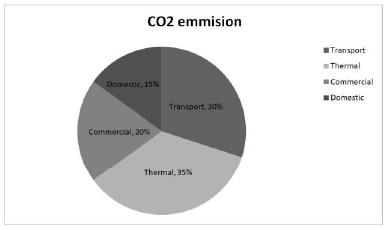Q. Which of the following sectors together emit 4 mm of CO2 every year?

Solution:

Total emission is 8. And 4 mm is 50% (half) of 8 (total emission)
From the given circle, transport + commercial, represent half circle (50%) Similarly (Thermal + Domestic) is also 50%. But it is not given in options.
So, the answer is (c): Transport +
Commercial.

QUESTION: 16

Directions for: Examine the information given in the following paragraph and answer the items there follow:

A school is celebrating Sport week in which five games viz. Cricket, Badminton, Tennis, Football and Volleyball are to be held in five different days in a week from Monday to Friday. Only one sport can be played on each day. Cricket cannot be played on Tuesday. Players for Badminton is available only on Tuesday. Volleyball is played immediately after the day of Cricket. Football has to played immediately before the day of Cricket.

Q. Which sport is the last one in the week?

Solution:

Badminton is on Tuesday. Cricket is on Thursday because Volleyball has to be immediately after that (Friday) and Football immediately before Cricket (Wednesday). Remaining, Tennis subject is on Monday.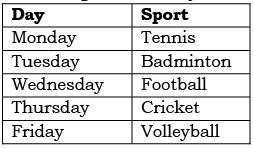QUESTION: 17

In a group of 20 children, two children have hundred marbles each and three do not possess any marbles. The average number of marbles with the rest of the children is 40. What is the average number of marbles with the group of children?

Solution:

Total number of marbles with the children = (100 × 2) + (40 × 15) + (3 × 0)  = 800.
Average number of marbles with the children = Total number of marbles/Total number of children = 800/20 = 40

QUESTION: 18

Direction: The total annual CO2 emissions from various sectors are 8 mm. In the Pie Chart given below, the percentage contribution to CO2 emissions from various sectors is indicated.Q. Which of the following sectors have emission difference of 0.8 mm between them?

Solution:

Total is 8 mmt (100%) So 0.8 mmt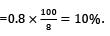Find out which two sectors have a % difference of 10%, Transport minus Commercial = 30 – 20 = 10%. So (b) is the answer.

QUESTION: 19

If = stands for ÷; + stands for -; - stands for +; × stands for =; ÷ stands for ×; which of the following is true?

Solution:

Substituting the signs equation 1 becomes: 8 × 4 + 1 ÷ 1 - 1 = 32, which is correct.

QUESTION: 20

The whole biosphere, like the individual organisms that live inside it, exists in a chemically dynamic state. In this homeostatic system, a great number of organic compounds are synthesized, transformed, and decomposed continuously; together, these processes constitute the major parts of the carbon cycle. For the smooth operation of this cycle, degradation is just as important as synthesis: the green plants produce great quantities of polymers, such as cellulose, and innumerable other compounds like alkaloids, terpenes, and flavonoids, that green plants cannot use as sources of energy during respiration. The release of the carbon in these compounds for recycling depends almost entirely on the action of both aerobic and anaerobic bacteria and certain types of fungi. Some bacteria and fungi possess the unique and extremely important biochemical asset of being able to catalyse the oxidation of numerous inert products, thereby initiating reaction sequences that produce carbon dioxide and so return much carbon to a form that actively enters into life cycles once again.

Q. The passage contains information that would answer which of the following questions about the carbon cycle?
1. What are some of the compounds that are broken down in the carbon cycle?
2. Why are some compounds that are involved in the carbon cycle less reactive than others? 3. What role do bacteria and fungi play in the carbon cycle?

Solution:

In the passage it is information about the compounds that are broken down in the carbon cycle and the role bacteria and fungi play in the carbon cycle.

QUESTION: 21

The whole biosphere, like the individual organisms that live inside it, exists in a chemically dynamic state. In this homeostatic system, a great number of organic compounds are synthesized, transformed, and decomposed continuously; together, these processes constitute the major parts of the carbon cycle. For the smooth operation of this cycle, degradation is just as important as synthesis: the green plants produce great quantities of polymers, such as cellulose, and innumerable other compounds like alkaloids, terpenes, and flavonoids, that green plants cannot use as sources of energy during respiration. The release of the carbon in these compounds for recycling depends almost entirely on the action of both aerobic and anaerobic bacteria and certain types of fungi. Some bacteria and fungi possess the unique and extremely important biochemical asset of being able to catalyse the oxidation of numerous inert products, thereby initiating reaction sequences that produce carbon dioxide and so return much carbon to a form that actively enters into life cycles once again.

Q. The author implies that which of the following is the primary reason that degradation is as important as synthesis to the smooth operation of the carbon cycle?

Solution:

From the passage it can be inferred that that degradation is as important as synthesis to the smooth operation of the carbon cycle as decomposition permits the recycling of carbon that would otherwise be fixed in certain substances.

QUESTION: 22

Based on the following bar graph. (Number of Industrial Townships in 1978 is 160000)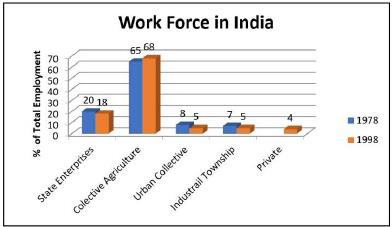Which was the first year when people were employed in private enterprises?

Solution:

Although we can definitely say that the people were employed in Private Enterprises after 1978 and before or in 1998, we do not have data prior to 1978 and intermediate years’ data. So, cannot be determined.

QUESTION: 23

Based on the following bar graph. (Number of Industrial Townships in 1978 is 160000)If it is known that the total work force in India increases at a simple rate of 10% per annum, then by what per cent does the number of people employed in the Urban Collectives change?

Solution:

Let total work force in 1978 be ‘n’. Then in 1998, it will be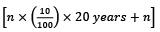People employed in urban collectives in 1978 = 0.08n, in 1998 = 0.05 × 3n = 0.15n.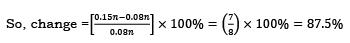QUESTION: 24

Directions
A book is divided into 6 chapters. The following pie chart denotes the percentage of the total pages devoted to each chapter.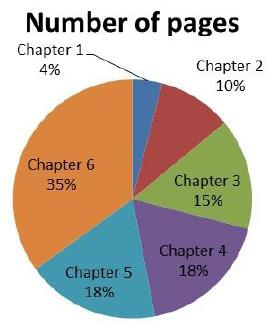If Chapter 1 contains 60 pages, the number of pages in chapter 5 is?

Solution:

Chapter 5 is 18%, whereas Chapter 1 is 4%.
Chapter 5 is 4.5 times Chapter 1.
So, number of pages in Chapter 5 = 4.5 × Number of pages in chapter 1 = 4.5 × 60 = 270.

QUESTION: 25

Directions

A book is divided into 6 chapters. The following pie chart denotes the percentage of the total pages devoted to each chapter.The central angle for Chapter 6 is x degrees more than that of chapter 5. The value of x is

Solution:

Angle for Chapter 6 = (35/100) × 360° = 126°
Angle for Chapter 5 = (18/100) × 360° = 64.8°
Required difference = 61.2°

QUESTION: 26

Four friends A, B, C and D planned a get-together at D's home. The houses of A and B face each other on a road going north-south, A's being on the western side. A comes out of his house, turns left, travels 5 km. turned right, travels 5 km to the front of D's house. B does exactly the same and reaches the front of C's house. In this context, which one of the following statements is correct?

Solution:

Given information diagrammatically can be shown as follows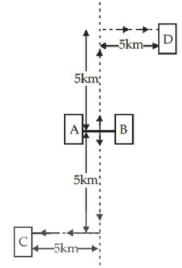From the above diagram, it is clear that the houses of C and D are less than 20 km apart.

QUESTION: 27

In the Venn diagram given below, what is the total number of singers who are only painters or only dancers but not both painter as well as dancer.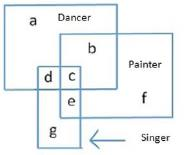Solution:Singers who are dancers but no painters = d
Singers who are painters but not dancers = e
So, the total number of singers who are only painters or only dancers but not both painter as well as dancer = d + e

QUESTION: 28

The table represent the percentage expenditure of the income of A, B, C, D, E and F on different items.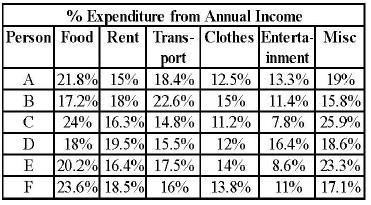If the annual income of B and C are ₹2,16,000 and ₹2,64,000 respectively, what is the difference between the amount spent by them on transport?

Solution: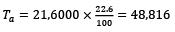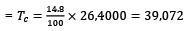= Difference = 48,816 – 39,072= ₹9,744

QUESTION: 29

The table represent the percentage expenditure of the income of A, B, C, D, E and F on different items.If the amount of money spent on food by C and D are ₹72000 and ₹86400 respectively, then the annual income of C is what percentage of the annual income of D?

Solution:

The correct option is C.
24% of total of C = 72000
Total of C = 72000*100/24 = 300000
18% of D = 86400
Total of D = 86400*100/18 =480000
Percentage of C with respect to D = 300000/480000*100 = 62.5%

QUESTION: 30

The table represent the percentage expenditure of the income of A, B, C, D, E and F on different items.The percentage of amount of money spent by E on entertainment is what percentage of the amount of money spent by F on transport?

Solution:

Eentertainment = 8.6%
FTransport = 16%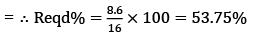QUESTION: 31

Road the passage given below and the two statements that follow (given on the basis of the passage);

Four athletes are waiting at Beijing airport for a Mumbai flight. Two are Sprinters and the other two are Badminton players. Two are from Maharashtra and two are from Bengal. No two of the same profession are from the same state. Two are Muslims and two are Christian. No two of the same religion are of the same profession nor do they are from the same state, The Bengal’s Sprinter is a Christian.
1. The Christian-Badminton player is from Maharashtra.
2. The Maharashtra’s Sprinter is a Muslim.

Q. Which of the above statement(s) is/are correct conclusion(s)?

Solution:

The correct option is C.
Sprinter =

1. Bengal - christian
2. Maharashtra - muslim

1. Bengal - muslim
2. Maharashtra - christian
QUESTION: 32

10% of α is 5 times 20% of β. The ratio β: α is:

Solution:

10% of α is 5 times 20% of β
Or
(10/100) α = 5 × (20/100) β
Or
0.1α = 5 × 0.2 β
Or β/ α =1/10

QUESTION: 33

Refer to the following graph related to the percentage of persons over 25 years of age and with more than 4 years college education living in country X.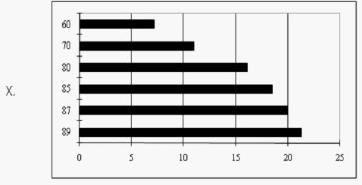What percentage of persons of 25 and over completed 4 years of college or more in 1970?

Solution:

By visual observation, 11% of person of 25 and over completed 4 years of college or more in 1970.

QUESTION: 34

At what time between 5 o'clock and 6 o'clock, both the needles of a clock will coincide each other?

Solution:

At 5 o' clock, the hands are 25-minute spaces apart.
Hence minute hand needs to gain 25 more-minute spaces over the hour hand so that the hands will coincide each other.
In 60 minutes, minute hand gains 55-minute spaces over the hour hand.
Hence, time taken for gaining 25-minute spaces by minute hand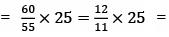300/11 minutes
Hence hands will coincide at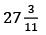minute past 5.

QUESTION: 35

A room is 15m 25 cm long and 9m 25 cm wide. The room has to be tiled using square tiles of side 41 cm. If no tile has to be cut and maximum area possible is covered, how many tiles can be placed in the room?

Solution:

1525/41 = 37.2
Along the length, 37 tiles can be placed without cutting any tile. 925/41 = 22.5
Along the breadth, 22 tiles can be placed without breaking any tile.
(After placing all these tiles some area will remain uncovered)
Maximum number of tiles that can be used = 37 × 22 = 814.

QUESTION: 36

Refer to the following graph related to the percentage of persons over 25 years of age and with more than 4 years college education living in country X.What was the average percentage increase per year from 1970 to 1980?

Solution:

%age in year 1980 = 16.5. %age in year 1970 = 11%. Percentage increase = (16.5 - 11)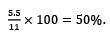Average % age increase over 10 years = 50/10 = 5%

QUESTION: 37

A, B and C have a few coins with them. 7 times the number of coins that A has is equal to 5 times the number of coins B has while 8 times the number of coins B has is equal to 13 times the number of coins C has. What is the minimum number of coins with A, B and C put together?

Solution: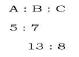So, final ratio  of [A:B:C :: (5 x 13) : (7 x 13) : (8  x 7)]
minimum number of coins is equal to sum of their ratio  = (65 + 91 + 56) = 212

QUESTION: 38

Directions: In an examination of 150 marks, all questions carry equal marks. 3 marks are awarded for every correct answer and 2 marks are deducted for every wrong answer. All the questions are compulsory and one mark is deducted for every unanswered question.

Q. A student answered 45 questions. Which of the following cannot be the marks obtained by the student?

Solution:

Each question carries 3 marks.
Number of questions = 150/3 = 50.
5 unattempted questions fetch the student negative 5 marks
Assuming no question was wrong → Marks obtained by student = 45 × 3 – 5 = 130
For every wrongly answered question, the marks obtained will drop by 5.
So, marks obtained will always be a multiple of 5.

QUESTION: 39

A shopkeeper sells an article at a loss of 25%, but when he increases the selling price by Rs. 300 he earns a profit of 5% on the cost price if he sells same article at Rs.1250, what is her profit percentage?

Solution: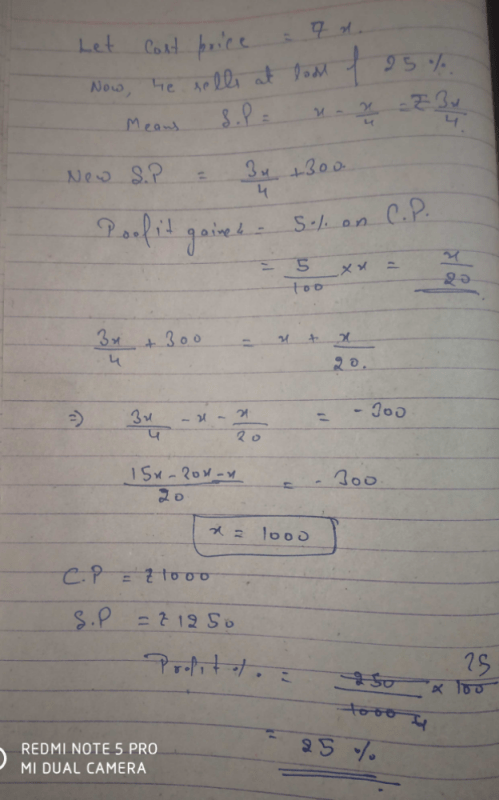QUESTION: 40

Refer to the following graph related to the percentage of persons over 25 years of age and with more than 4 years college education living in country X.If in 1987, a typical state had 125,000 persons of 25 and over with 4 years of college or more. What population of the state was 25 and over?

Solution:

In 1987, 20% of persons and over with 4 years of college and more i.e. 1,25,000 persons(given).
20% of population in 1987 = 1, 25,000. Total population = 100 x 125000 / 20 = 6,25,000

QUESTION: 41

Directions: In an examination of 150 marks, all questions carry equal marks. 3 marks are awarded for every correct answer and 2 marks are deducted for every wrong answer. All the questions are compulsory and one mark is deducted for every unanswered question.

Q. ​A group of students appeared for the exam. Each of them attempted 45 questions and each of them obtained different marks. If none of the students scored negative marks, how many students may have appeared for the exam?

Solution:

As discussed above, the maximum marks that can be obtained in this condition is 130.
For a single wrong answer, marks will get reduced by 5.
No student obtained negative marks. So, the marks obtained may be 0, 5, 10, ...., 130; i.e. 27 possible marks.
So, 27 students can appear for the exam and each of them may get a different score.

QUESTION: 42

Consider the following statements;
P + Q means P is brother of Q
P – Q means P is sister of Q
P × Q means P is husband of Q
P ÷ Q means P is wife of Q

Q. Then which of the following means C is sister-in-law of A?

Solution:

C is sister-in-law of A means C is her husband’s sister therefore only option (d) satisfy all these conditions.

QUESTION: 43

An old lady spent one twelfth of her life as a child and one seventh was spent as a teenager. One sixth of her life was spent between the time she became an adult and the time she married. Three years after marriage her daughter was born and the daughter died six years before she died. She lived to be twice as old as her daughter did. How long did the lady’s daughter live?

Solution:

Let the age of the lady at the time of her death be X.
Age spent as child = X/12
Age spent as a teenager = X/7
Age spent between adulthood and marriage = X/6
Age of daughter = X – (X/12 + X/7 + X/6 + 3 + 6) = X - 33X/84 – 9 = 51X/84 – 9
Now, 2(51X/84 – 9) = X
Or 18X/84 = 18
Or X = 84.
Age of daughter = X/2 = 42 years

QUESTION: 44

Refer to the following graph related to the percentage of persons over 25 years of age and with more than 4 years college education living in country X.What was the percent increase in the percentage of persons of 25 and over completing 4 years of college or more between 1960 and 1989?

Solution:

%age in year 1960 = 7%, %age in year 1989 = 21%, %age increase = 100 x (21 - 7) / 7 = 200% (approx.)

QUESTION: 45

Allen and Wolkowitz’s research challenge the common claim that homework—waged labour performed at home for a company— is primarily a response to women worker’s needs and preferences. By focusing on a limited geographical area in order to gather in-depth information, the authors have avoided the methodological pitfalls that have plagued earlier research on homework. Their findings disprove accepted notions about homeworkers: that they are unqualified for other jobs and that they use homework as a short-term strategy for dealing with child care. The authors conclude that the persistence of homework cannot be explained by appeal to such notions, for, in fact, homeworkers do not differ sharply from other employed women. Most homeworkers would prefer to work outside the home but are constrained from doing so by lack of opportunity. In fact, homework is driven by employer’s desires to minimize fixed costs: homeworkers receive no benefits and are paid less than regular employees.

Q. The passage is primarily concerned with

Solution:

From the passage we can conclude that the author is discussing research which opposes common claim.

QUESTION: 46

Allen and Wolkowitz’s research challenge the common claim that homework—waged labour performed at home for a company— is primarily a response to women worker’s needs and preferences. By focusing on a limited geographical area in order to gather in-depth information, the authors have avoided the methodological pitfalls that have plagued earlier research on homework. Their findings disprove accepted notions about homeworkers: that they are unqualified for other jobs and that they use homework as a short-term strategy for dealing with child care. The authors conclude that the persistence of homework cannot be explained by appeal to such notions, for, in fact, homeworkers do not differ sharply from other employed women. Most homeworkers would prefer to work outside the home but are constrained from doing so by lack of opportunity. In fact, homework is driven by employer’s desires to minimize fixed costs: homeworkers receive no benefits and are paid less than regular employees.

Q. According to the passage, which of the following has been generally believed about homework?

Solution:

In the passage it is mentioned “common claim that homework—waged labour performed at home for a company—is primarily a response to women worker’s needs and preferences.” implies that general belief is that homework is primarily a response to the preferences of women workers.

QUESTION: 47

Allen and Wolkowitz’s research challenge the common claim that homework—waged labour performed at home for a company— is primarily a response to women worker’s needs and preferences. By focusing on a limited geographical area in order to gather in-depth information, the authors have avoided the methodological pitfalls that have plagued earlier research on homework. Their findings disprove accepted notions about homeworkers: that they are unqualified for other jobs and that they use homework as a short-term strategy for dealing with child care. The authors conclude that the persistence of homework cannot be explained by appeal to such notions, for, in fact, homeworkers do not differ sharply from other employed women. Most homeworkers would prefer to work outside the home but are constrained from doing so by lack of opportunity. In fact, homework is driven by employer’s desires to minimize fixed costs: homeworkers receive no benefits and are paid less than regular employees.

Q. Allen and Wolkowitz’s research suggests that each of the following is true of most homeworkers EXCEPT:

Solution:

Allen and Wolkowitz’s research suggest that homeworker perform professional-level duties rather than manual tasks or piecework.

QUESTION: 48

The data charges of Airtel is Rs. 90 per day. The corresponding charges of Idea are 10% lower than that of Airtel. A person uses Airtel for the first fortnight of a month and Idea for the second fortnight. Had he used Idea for the whole of the month, what percentage of his expenses on data for that month would he had saved (approximately)?

Solution:

Charge for Airtel for 15 days = 15 × 90 = Rs 1350
Charge for Idea for 15 days = 1350 – 10% of 1350 = 1350-135 = Rs 1215
Total monthly expense on data = 1350 + 1215 = Rs 2565
Required percentage = (135/2565) × 100 = 5.2 % (approx)

QUESTION: 49

Refer to the following graph related to the percentage of persons over 25 years of age and with more than 4 years college education living in country X.Q. If some other country had three times as many people of 25 and over in 1989 as the country X with the same number of persons completing 4 years of college or more, what percentage of that country’s people of 25 and over would complete college?

Solution:

If country X had 100 people over 25 in year 1989, then 21 were educated. The other country has 300 people over 25.
The number of educated is still 21.
So, as a %age that works out to 21/300 × 100 = 7%.

QUESTION: 50

Direction: During the last summer vacation, Ankur went to a Shimla where he took part in swimming and boating. This summer, he is looking forward to a trip to Ooty where he hopes to hike, dance and learn to play cricket.

Q. Based on the above information, four conclusions, as given below, have been made. Which one of these logically follows from the information given above?

Solution:

No reasons are specified in the question of going to different places. As Ankur has been going to different places in the past two years, it can be said that he goes to some place every summer.

QUESTION: 51

Directions: Education and gender are strong indicators of life expectancy. Men and women with a tertiary education, on average, live significantly longer than those of the same gender who are less educated; the widest gap, however, is between tertiary-educated men and their less-educated counterparts.

Q. ​What does the above passage imply?

Solution:

(a) is incorrect as the passage only talks about the role of education and gender in life expectancy and the comparison between male and female natural health has not been made in the passage. Hence, it cannot be inferred from the passage.
(b) is incorrect as it is an extreme statement and the passage only states that it is a strong indicator of life expectancy.
(c) is incorrect as gender discrimination has not been discussed in the passage.
(d) is correct as the passage states how the widest gap in life expectancy is between tertiary educated men and less educated women. Logically, the widest gap is between the highest and the lowest. Hence, less educated women have least life expectancy.

QUESTION: 52

The following piechart shows the percentage distribution of the expenditure incurred in publishing a book. Study the pie-chart and the answer the questions based on it.
Various Expenditures (in percentage) Incurred in Publishing a Book.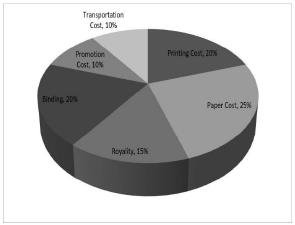If for a certain quantity of books, the publisher has to pay Rs. 30,600 as printing cost, then what will be amount of royalty to be paid for these books?

Solution:

Let the amount of Royalty to be paid for these books be Rs. r
Then, 20:15 = 30600:r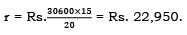QUESTION: 53

The following piechart shows the percentage distribution of the expenditure incurred in publishing a book. Study the pie-chart and the answer the questions based on it.
Various Expenditures (in percentage) Incurred in Publishing a Book.What is the central angle of the sector corresponding to the expenditure incurred on Royalty?

Solution:

Central angle corresponding to Royalty = 15% of 360º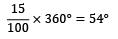QUESTION: 54

A man purchases two chairs A and B at total cost of Rs. 5,000. He sells A with 25% profit and B with 16.66% loss and get the same selling price for both the chairs. What are the purchasing price of A and B respectively?

Solution:

Let the cost price of A and B be “a” and (5,000 - a) respectively.
Selling price of A = Selling price of B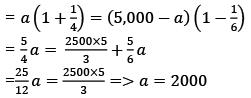So, purchasing price of A and B are a = Rs.2000 and (5000-a) = Rs. 3000

QUESTION: 55

Direction: The Fourth Industrial Revolution (IR) has the transformational power to unlock economic value that was previously inaccessible, by decoding nature’s DNA and by learning from its functions and processes. If the dividends are shared equitably, an inclusive bioeconomy could be created that provides a significant new funding stream for environmental conservation efforts.

Q. Which of the following is most rational inference from the above passage?

Solution:

(a) is incorrect as the passage does not talk about bio-economy replacing the present economic system as a whole.
(b) is correct as the passage discusses how the Fourth IR can help reap economic benefits of nature via research and with equitable distribution of these benefits, a bio-economy thus created will help fund environmental conservation efforts.
(c) is incorrect as the passage does not claim to secure funding of all conservation efforts, it only claims that it will help provide a significant flow of funds.
(d) is incorrect as the passage does not talk about the need of funding in the Fourth IR research works.

QUESTION: 56

The following pie chart shows the percentage distribution of the expenditure incurred in publishing a book. Study the pie-chart and the answer the questions based on it. Various Expenditures (in percentage) Incurred in Publishing a Book.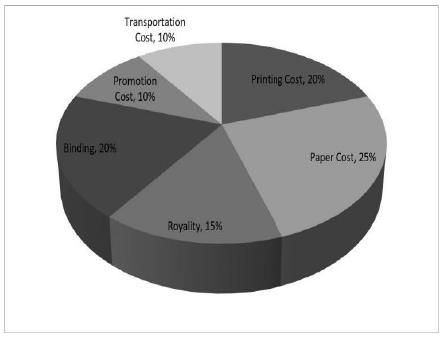The price of the book is marked 20% above the C.P. If the marked price of the book is Rs. 180, then what is the cost of the paper used in a single copy of the book?

Solution:

Clearly, marked price of the book = 120% of C.P.
Also, cost of paper = 25% of C.P
Let the cost of paper for a single book be Rs. n.
Then, 120: 25 = 180: n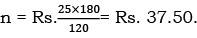QUESTION: 57

Price of diamond is directly proportional to square of its weight. A person broke down diamond in the ratio 5: 2: 7 and faces a loss of Rs 11800. Find initial price of diamond?

Solution:

Let the weight of the three pieces of the broken diamond = 5 gm, 2 gm and 7 gm respectively,
Then, weight of diamond before breaking = 14gm
Let cost of 1gm of diamond = Rs. ‘x’, Then, cost of diamond before breaking = Rs. (14)2x = Rs. 196x,
⇒ Cost of diamond after breaking = (52 + 22 + 72) x = 78x
⇒ Decrease in cost = 196x – 78x
According to the question:
⇒ 118x = 11800
⇒ x = 100.
Hence, initial price of diamond piece = 196x = 196 × 100 = 19600

QUESTION: 58

Direction: Violence in schools and other education settings causes serious harm and distress to children and adolescents that can last into adulthood. As the UN World Report on Violence against Children observed, it is a global phenomenon. Policies, laws and strategies to prevent school-related violence depend on accurate knowledge of its global prevalence, trends and effects, but such evidence is lacking.

Q. Which of the following is the most rational inference from the above passage?

Solution:

(a) is incorrect as it is an extreme statement with regards to prevention of violence in educational institutes which cannot be inferred from the passage.
(b) can be considered right as the passage does highlight the need of ending such violence but it isn’t the most rational inference from the passage.
(c) is incorrect as the passage does not comment on the importance of violence in adult distress.
(d) is correct as the passage talks about the ill effects of violence in educational settings and how lack of evidence on prevalence, trends and effects has hindered the policymaking in its prevention. Hence, the given statement can be inferred from the passage.

QUESTION: 59

The following piechart shows the percentage distribution of the expenditure incurred in publishing a book. Study the pie-chart and the answer the questions based on it. Various Expenditures (in percentage) Incurred in Publishing a Book.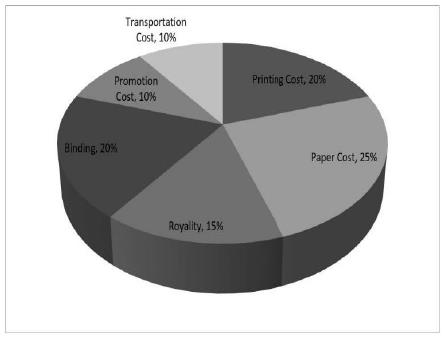If 5500 copies are published and the transportation cost on them amounts to Rs. 82500, then what should be the selling price of the book so that the publisher can earn a profit of 25%?

Solution:

For the publisher to earn a profit of 25%, S.P. = 125% of C.P.
Also, Transportation Cost = 10% of C.P.
Let the S.P. of 5500 books be Rs. x.
Then, 10 : 125 = 82500 : x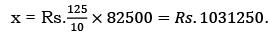S.P. of one book =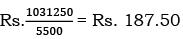QUESTION: 60

In a class 40% of the students enrolled for Math and 70% enrolled for Economics. If 15% of the students enrolled for both Math and Economics, what % of the students of the class did not enrol for either of the two subjects?

Solution:

We know that (A ∪ B) = A + B - (A ∩ B), where (A ∪ B) represents the set of people who have enrolled for at least one of the two subjects Math or Economics and (A ∩ B) represents the set of people who have enrolled for both the subjects Math and Economics.
(A ∪ B) = A + B - (A ∩ B)
⇒ (A ∪ B) = 40% + 70% - 15% = 95%
That is 95% of the students have enrolled for at least one of the two subjects Math or Economics.
Therefore, the balance (100 - 95) % = 5% of the students have not enrolled for either of the two subjects.

QUESTION: 61

Direction: Although the term “affordable housing” has been around for many years, the past two years have seen a renewed focus from the government to spur activity in the segment via various initiatives undertaken. While these initiatives have done enough to set the ball rolling, however, the growth momentum of the sector will be directly proportional to the proactive as well as reactive pace of the government. 20.

Q. Which of the following is most rational inference from the above passage?

Solution:

(a) is incorrect as the passage does not claim the inactivity of the government in the segment.
(b) is correct as the passage says “…done enough to set the ball rolling, however, the growth momentum of the sector will be directly proportional to the proactive as well as reactive pace of the government”.
(c) is incorrect as it guarantees the accomplishment of affordable housing and that too soon, but the passage does not endorse such claim and does not commit to future growth momentum.
(d) is incorrect as the passage does not mention the idea of input cost in affordable housing. Hence, it cannot be inferred from the passage.

QUESTION: 62

The following piechart shows the percentage distribution of the expenditure incurred in publishing a book. Study the pie-chart and the answer the questions based on it. Various Expenditures (in percentage) Incurred in Publishing a Book.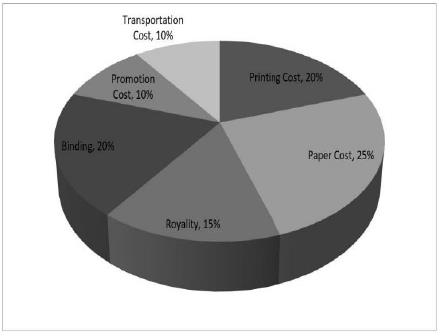Q. Royalty on the book is less than the printing cost by:

Solution:

Printing Cost of book = 20% of C.P.
Royalty on book = 15% of C.P. Difference = (20% of C.P.) - (15% of C.P) = 5% of C.P.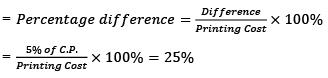QUESTION: 63

One day, Ravi left home and cycled 20 Km southwards, turned right and cycled 10 km and turned right and cycled 20 Km and turned left and cycled 20 Km. How many kilometres will he have to cycle to reach his home straight?

Solution: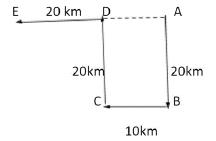Ravi starts from home at A, moves 20 Km in south to B. Then he turns right and moves 10 Km to C, then he turns right and moves 20 Km to D, then he turns lefts and moves 20 Km to E.
So, from image it is clear that, if he moves straight then he will have to move AD+DE, AD = BC = 10 Km.
So, he will have to move 10 + 20 = 30 Km.

QUESTION: 64

Direction: Despite a recent acceleration of global economic activity, potential output growth is flagging. At 2.5 percent in 2013-17, post-crisis potential growth is below its longer-term average with an even steeper decline in emerging market and developing economies. This slowdown is evidence of slowing productivity growth and demographic trends that dampen labour supply growth.

Q. Which among the following is the most logical, rational and critical inference that can be made from the above passage?

Solution:

(a) is correct since the passage first tells how rising activity hasn’t resulted in high output growth and then tells what this situation points to, which is slowing productivity growth and labour supply growth.
(b) is incorrect as the passage does not tell that output is falling, it only states that the output growth is falling.
(c) is incorrect as it completely gives up hope on the emerging and developing economies which cannot be sufficiently deduced from the passage.
(d) is incorrect as the passage does not talk about skilling and redistribution of labour. Hence it is beyond the passage’s scope.

QUESTION: 65

Study the following graph and answer the questions that follow.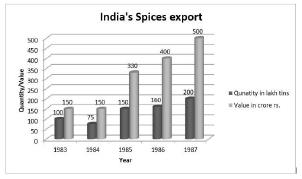​In which year was the value per tin the minimum?

Solution:

In 1983, value per tin is 150/100, which is the lowest for all the years.

QUESTION: 66

Study the following graph and answer the questions that follow.If in 1986 tins were exported at the same rate per tin as in 1985, then what would be the value of exports in 1986? (Crores of Rupees)

Solution:

Value in 1985: 330/150 = 2.2
Value in 1986: 160 x 2.2 = 352.

QUESTION: 67

Statement: Over 27,000 bonded labours identified and freed are still awaiting rehabilitation.
Courses of action:
1. More cases of bonded labour identified.
2. Till the rehabilitation facilities are available, the bonded labours should not be freed.
3. The implementation in the way of speedy and proper rehabilitation of bonded labours should be removed.

Solution:

A feasible and effective course to combat the problem is only the (3) action plan. It impediments in the way of speedy and proper rehabilitation should be removed.

QUESTION: 68

Direction: The Indian government’s efforts to make business and commerce easy have been widely acknowledged. The next frontier on the ease of doing business is addressing pendency, delays and backlogs in the appellate and judicial arenas which are not only hampering dispute resolution and contract enforcement, discouraging investment, stalling projects, hampering tax collections but also stressing tax payers, and escalating legal costs.

Q. Which of the following is most logical and rational inference from the above passage?

Solution:

(a) is incorrect as the passage does not present the scope of doing away with judicial interference in business and commerce.
(b) is correct as the passage presents the need for efforts to address judicial and appellate pendency in order to improve the already acknowledged ease of doing business of India. Hence the given statement can be logically inferred from the passage.
(c) is incorrect as the passage asks for further improvement in the ease of doing business arena by addressing the judicial pendency.
(d) is incorrect as fast track courts and separate tribunals have not been discussed in the passage.

QUESTION: 69

Go through the following pie chart and answer the questions given below that.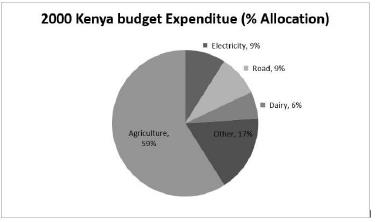Q. ​Approximately how many degrees should be there in the central angle of the sector for agriculture expenditure?

Solution:

In a pie chart, 100% is spread over 360°. Therefore 1 % = 3.6°.
Agriculture expenditure = 59 %. Therefore 3.6×59 = 212.4°.

QUESTION: 70

Go through the following pie chart and answer the questions given below that.Q. Approximately what is the ratio of expenditure on agriculture to that on dairy?

Solution:

Budget expenditure is proportional to % of area covered, ratio of agriculture to dairy expenditure would be ratio of corresponding % allocations. Therefore, Agriculture/Dairy = 59/6 = 10/1.

QUESTION: 71

Theorists are divided concerning the origin of the Moon. Some hypothesize that the Moon was formed in the same way as were the planets in the inner solar system (Mercury, Venus, Mars, and Earth)—from planet-forming materials in the presolar nebula. But, unlike the cores of the inner planets, the Moon’s core contains little or no iron, while the typical planet-forming materials were quite rich in iron. Other theorists propose that the Moon was ripped out of the Earth’s rocky mantle by the Earth’s collision with another large celestial body after much of the Earth’s iron fell to its core. One problem with the collision hypothesis is the question of how a satellite formed in this way could have settled into the nearly circular orbit that the Moon has today. Fortunately, the collision hypothesis is testable. If it is true, the mantle rocks of the Moon and the Earth should be the same geochemically.

Q. The primary purpose of the passage is to

Solution:

In the passage author present two hypotheses concerning the origin of the Moon.

QUESTION: 72

Theorists are divided concerning the origin of the Moon. Some hypothesize that the Moon was formed in the same way as were the planets in the inner solar system (Mercury, Venus, Mars, and Earth)—from planet-forming materials in the presolar nebula. But, unlike the cores of the inner planets, the Moon’s core contains little or no iron, while the typical planet-forming materials were quite rich in iron. Other theorists propose that the Moon was ripped out of the Earth’s rocky mantle by the Earth’s collision with another large celestial body after much of the Earth’s iron fell to its core. One problem with the collision hypothesis is the question of how a satellite formed in this way could have settled into the nearly circular orbit that the Moon has today. Fortunately, the collision hypothesis is testable. If it is true, the mantle rocks of the Moon and the Earth should be the same geochemically.

Q. According to the passage, Mars and the Earth are similar in which of the following ways?
1. Their satellites were formed by collisions with other celestial bodies.
2. Their cores contain iron.
3. They were formed from the presolar nebula.

Solution:

From the passage choice (2) and (3) is observed.

QUESTION: 73

Consider the figure given below: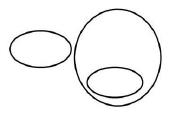​The figure represents the relationship between which of the following sets correctly?

Solution:

Option (a): Tea and Coffee both are subset of beverages. Picture does not represent it.
Option (b): Authors and Teachers may be men or women. So, both will have a common part with men. The picture given does not represent it.
Option (c): Boys, students and athletes will have boys as common. The picture given does not represent it.
Option (d): Sparrows are a subset of birds and mice have nothing in common with birds. The picture represents the same.

QUESTION: 74

Go through the following pie chart and answer the questions given below that.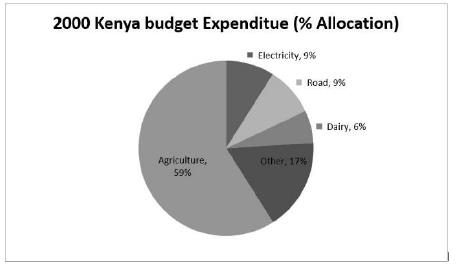In Kenya, in 2000, a total expenditure of Rs. 120mn was incurred. Approximately how many million did the Kenya government spend on roads?

Solution:

Total expenditure = 100% = Rs. 120 mn. Expenditure on roads = 9% = 9/100 × 120 =
Rs. 10.8mn.

QUESTION: 75

In a school head volunteer is to be selected among the students of four classes class 7, class 8, class 9 and class 10. The formula agreed by them was the acceptance of a student by most of the classes. Four students, Ankit, Binod, Chinta and Damini approached the classes for that position.
Ankit is acceptable to Class 7 but not to class 10.
Binod is acceptable to class 9 but not to class 8.
Chinta is acceptable to class 7 and class 9.
J
Damini is acceptable to class 7 and class 8.

Q. When Binod is preferred by Class 7 and Class 10 candidate, Chinta is preferred by class 8 and class 10, and candidate Ankit is acceptable to class 8 but not to class 9; who will be the head volunteer?

Solution:

Chinta was preferred by class 8 and class 10 and acceptable to class 7 and class 9. Therefore, Chinta will be head volunteer.

QUESTION: 76

The permutations of the word CLOCK are arranged alphabetically. Which of the following permutations will come first?

Solution:

The first permutation will have all the alphabets arranged in ascending order. In this order CCKLO comes first.

QUESTION: 77

Go through the following pie chart and answer the questions given below that.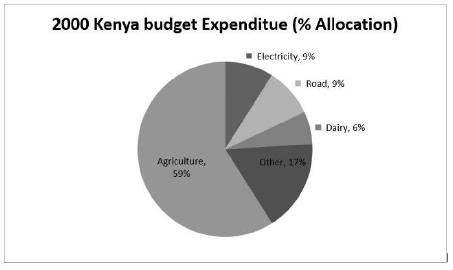If Rs. 9mn were spent in 2000 on Dairy, what would have been the total expenses in that year in million?

Solution:

9mn were spent on dairy. This amount represents 6% of total expenditure in the year
2000.
6 = (Dairy expenditure/Total expenditure) × 100
6 = (9/Total expenditure) × 100
Total Exp = 100 × (9/6) = Rs. 150mn.

QUESTION: 78

A earns 20% more than B. C earns 25% more than A. A earns 20% more than D. E earns 10% more than A. A, B, C, D, and E earn integer amounts less than Rs.100. What is the total amount earned by all five of them put together?

Solution:

Let the earnings be A, B, C, D, E.
From given conditions,
B = 5/6 A, C = 5/4 A, D = 5/6 A and E = 11/10 A
Taking LCM, A= 60.
Now, E = 60, B = 50, C = 75, D = 50 and E = 66
Adding A + B + C + D + E = 60 + 50 + 75 + 50 + 66 = 301.
Total amount earned by all 5 is 301 Rs.

QUESTION: 79

In a row of students, Raman is standing in fifth position from either end. Choose the statement that is correct regarding the students.

Solution:

Ram is fifth from both ends. Clearly, there are 9 students in the row.
9 students may be re-arranged into 3 rows of 3 students each.

QUESTION: 80

Go through the following pie chart and answer the questions given below that.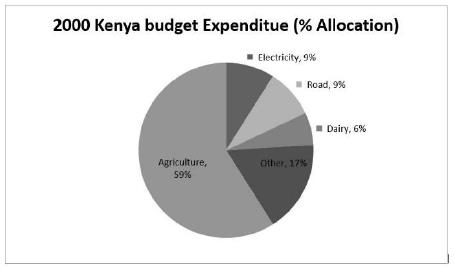In 1999, Kenya spent 11% of all its expenses on Roads. “Did Kenya spend more on Roads expenditure in 1999 than in 2000?” To answer the question, we

Solution:

% gives us just a proportional indication of total quantity.
Unless total expenditure is known, % of that is meaningless.
Hence, to compare expenditure on roads in 1999 and 2000, the total amount spent must be known.